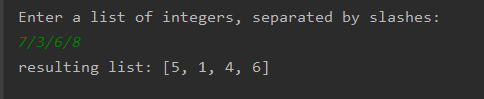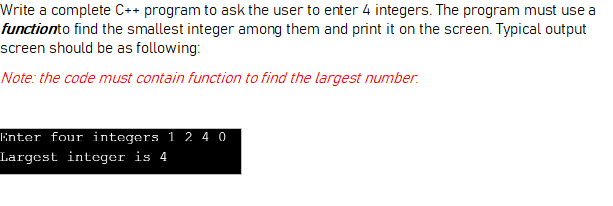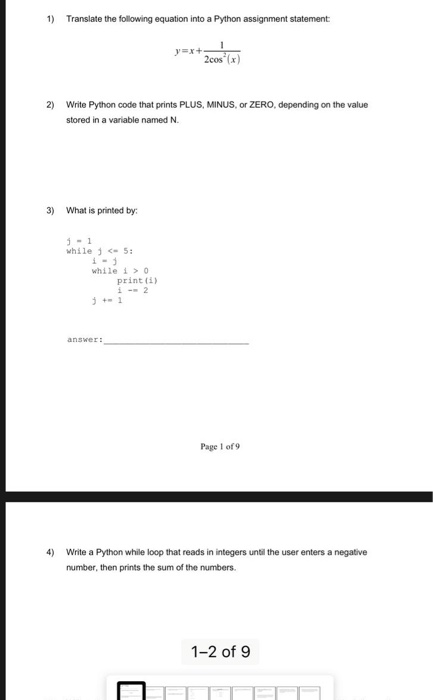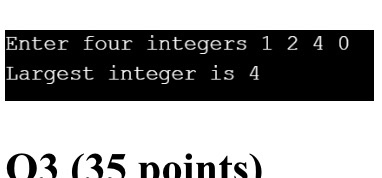Question

# write in python Create a program that will ask the user for a list of integers,...

write in python

Create a program that will ask the user for a list of integers, all on one line separated by a slash. construct a list named "adj_numbers" which contains the users input numbers after subtracting 2 from each number. then print the resulting list. the input statement and print statement are given to you in the starting code. a sample run of the program is as follows:

Enter a list of integers, separated by slashes:

7/3/6/8

resulting list: [5,1,4,6]

integer_string = input("Enter a list of integers, separated by slashes:\n")

```integer_string = input("Enter a list of integers, separated by slashes:\n")
lst = []
for word in integer_string.split('/'):
lst.append(int(word) - 2)
print("resulting list:", lst)
```#### Earn Coins

Coins can be redeemed for fabulous gifts.

Similar Homework Help Questions
• ### in python 3 Create a list or tuple with 5 different integers. Prompt the user for...

in python 3 Create a list or tuple with 5 different integers. Prompt the user for an integer. If the number is in the list (or tuple) print a message stating the number was found. Otherwise, print a message stating the number was not found. Assume the list includes the numbers 0, 2, 4, 6, 8, and 10.

• ### Write a complete C++ program to ask the user to enter 4 integers. The program must...Write a complete C++ program to ask the user to enter 4 integers. The program must use a function to find the smallest integer among them and print it on the screen. Typical output screen should be as following: Write a complete C++ program to ask the user to enter 4 integers. The program must use a functionto find the smallest integer among them and print it on the screen. Typical output screen should be as following: Note: the code...

• ### USING PYTHON Allow a user to input a comma-separated series of integers or floats. Create a...

USING PYTHON Allow a user to input a comma-separated series of integers or floats. Create a tuple sorted from lowest to highest value out of the input, then print it back to the user.

• ### urgent Help needed in python program ! Thanx # This is a function definition. You can...

urgent Help needed in python program ! Thanx # This is a function definition. You can ignore this for now. def parse_integer_list_from_string(string): """ Returns a list of integers from a string containing integers separated by spaces. """ # Split the line into a list of strings, each containing a number. number_string_list = string.split() # Create an empty list to store the numbers as integers. numbers = [] # Convert each string to an integer and add it to the list...

• ### 1) Translate the following equation into a Python assignment statement 2) Write Python code that prints...1) Translate the following equation into a Python assignment statement 2) Write Python code that prints PLUS, MINUS, O ZERO, depending on the value stored in a variable named N. 3) What is printed by: 3 - 1 while 5: while 10 Print ) Page 1 of 9 4) Write a Python while loop that reads in integers until the user enters a negative number, then prints the sum of the numbers. 1-2 of 9 4) Write a Python while...

• ### Write a PYTHON program that allows the user to navigate the lines of text in a...

Write a PYTHON program that allows the user to navigate the lines of text in a file. The program should prompt the user for a filename and input the lines of text into a list. The program then enters a loop in which it prints the number of lines in the file and prompts the user for a line number. Actual line numbers range from 1 to the number of lines in the file. If the input is 0, the...

• ### Write a complete C++ program to ask the user to enter 4 integers. The program must...

Write a complete C++ program to ask the user to enter 4 integers. The program must use a function to find the LARGEST integer among them and print it on the screen. Typical output screen should be as following: Note: the code must contain function to find the LARGEST number. Enter four integers 1 2 4 0 Largest integer is 4

• ### Write a complete C++ program to ask the user to enter 4 integers. The program must...Write a complete C++ program to ask the user to enter 4 integers. The program must use a function to find the largest integer among them and print it on the screen. Typical output screen should be as following: Note: the code must contain function to find the largest number. Enter four integers 1 2 4 0 Largest integer is 4 03 (35 points)

• ### In Python 3, Write a program that reads a set of floating-point values. Ask the user...

In Python 3, Write a program that reads a set of floating-point values. Ask the user to enter the values, then print: The number of values entered. The smallest of the values The largest of the values. The average of the values. The range, that is the difference between the smallest and largest values. If no values were entered, the program should display “No values were entered” The program execution should look like (note: the bold values are sample user...

• ### write the solution of the program by python 3 language : I need the program using...

write the solution of the program by python 3 language : I need the program using list or string or loops (while and for) or if,elif,else : Alex got a sequence of n integers a1,a2,…,an as a birthday present. Alex doesn't like negative numbers, so he decided to erase the minus signs from all negative numbers. As a result, he got a sequence of non-negative numbers. Print the resulting sequence. For example, if the sequence is  1, 5, -3, 4,...